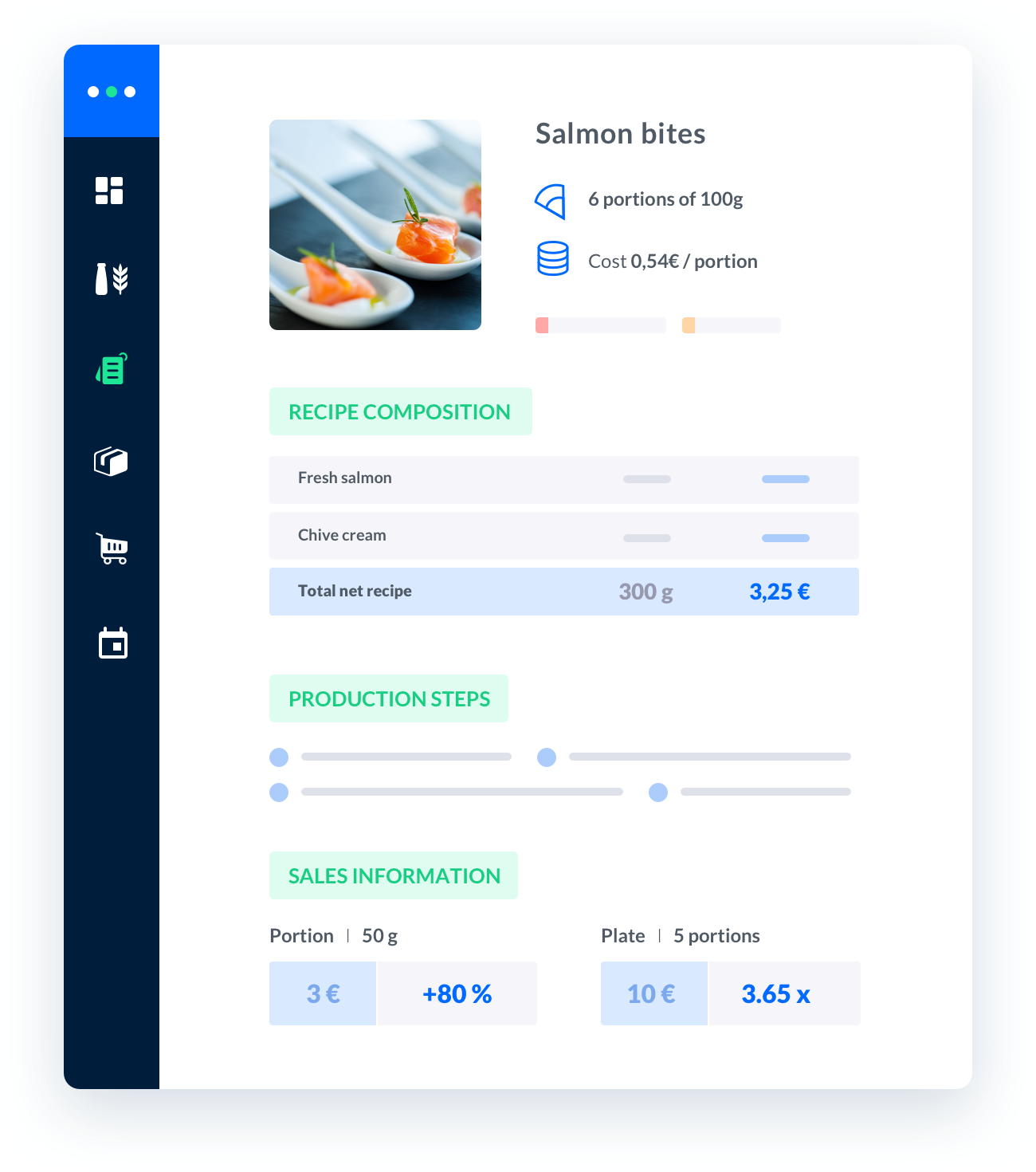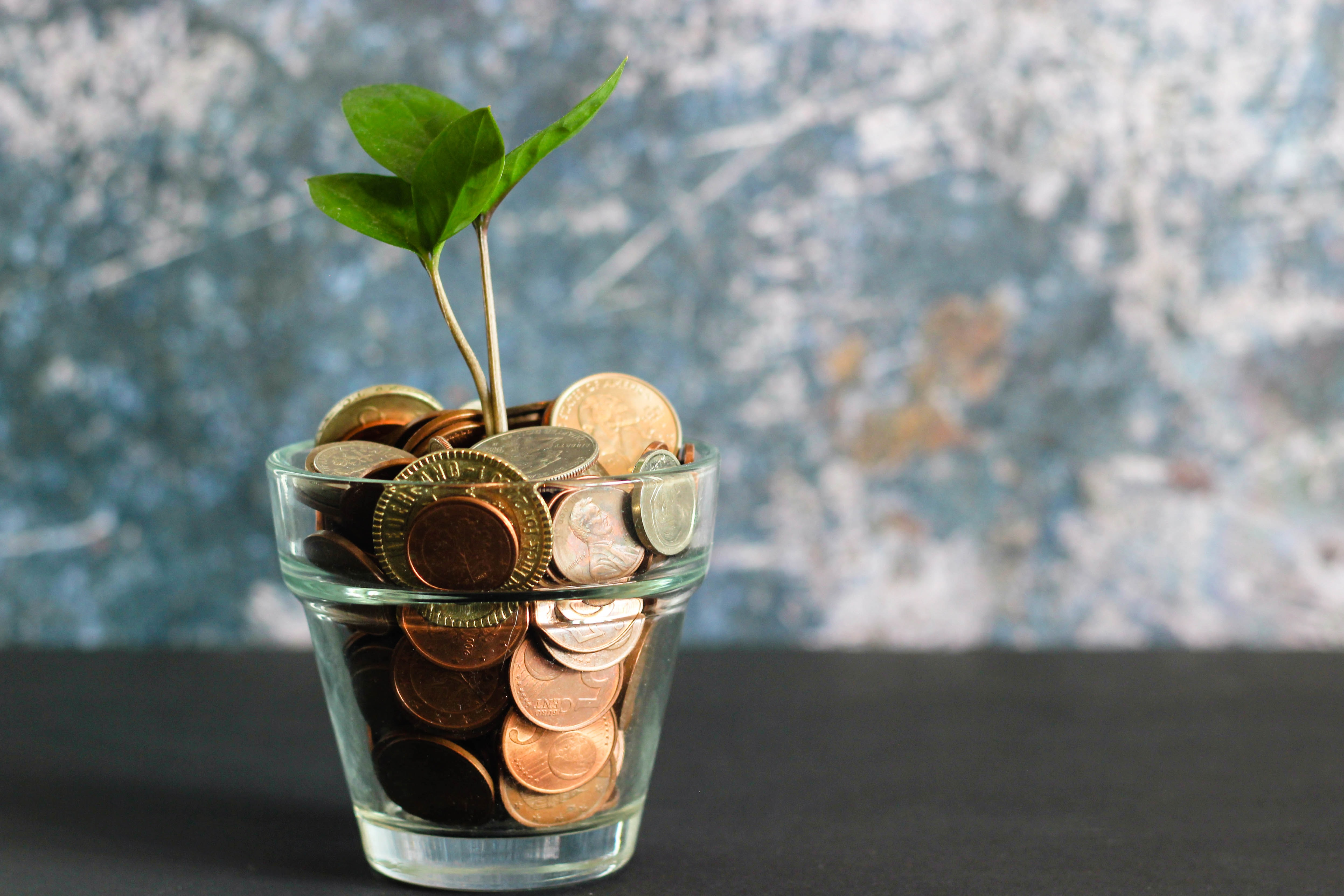# how to calculate cost percentage

Calculating cost percentage is an essential task for professionals in various industries, including the catering industry.It helps businesses determine their expenses and profitability, enabling them to make informed decisions and optimize their operations.

Published on 01/01/2023Sébastien Vassaux##### Melba: the food cost app to optimize the profitability of your restaurant

Discover how to optimize the profitability of your restaurant with melba##### The ultimate guide to food cost restaurant

Calculating cost percentage is an essential task for professionals in various industries, including the catering industry. It helps businesses determine their expenses and profitability, enabling them to make informed decisions and optimize their operations. In this comprehensive guide, we will explore the step-by-step process of calculating cost percentage, providing valuable insights and real-world examples to facilitate your understanding.

## Understanding Cost Percentage

Before delving into the calculation process, it is crucial to grasp the concept of cost percentage. Cost percentage refers to the proportion of expenses incurred in relation to the revenue generated. It is often expressed as a percentage and is used to assess the efficiency and profitability of a business.

### Types of Costs

Prior to calculating the cost percentage, it is important to identify and categorize the different types of costs involved in your catering business. Here are three common cost categories:

• Fixed Costs: These costs remain constant regardless of the level of production or sales. Examples include rent, insurance, and salaries of permanent staff.
• Variable Costs: Variable costs fluctuate with the level of production or sales. These expenses include raw materials, utilities, and wages for temporary staff.
• Semi-Variable Costs: Semi-variable costs have both fixed and variable components. An example is the cost of utilities, which may have a fixed monthly charge plus variable charges based on usage.

## Calculating Cost Percentage

### Step 1: Determine Total Costs

To calculate the cost percentage, you need to start by determining the total costs incurred within a specific timeframe. This includes all the costs associated with running your catering business, such as ingredients, labor, utilities, and overhead expenses.

### Step 2: Calculate Total Revenue

Next, you need to calculate the total revenue generated during the same timeframe. This includes all the income received from providing catering services, such as event fees, food sales, and any additional revenue streams.

### Step 3: Subtract Total Costs from Total Revenue

Subtracting the total costs from the total revenue gives you the gross profit. This represents the difference between the income generated and the expenses incurred.

### Step 4: Calculate the Cost Percentage

To calculate the cost percentage, divide the total costs by the total revenue and multiply the result by 100. This formula can be expressed as:

Cost Percentage = (Total Costs / Total Revenue) × 100

## Example Calculation

### Step 1: Determine Total Costs

Let's consider an example where the total costs incurred by a catering business over a month amount to \$10,000.

### Step 2: Calculate Total Revenue

During the same month, the catering business generated a total revenue of \$25,000.

### Step 3: Subtract Total Costs from Total Revenue

Subtracting the total costs of \$10,000 from the total revenue of \$25,000 gives a gross profit of \$15,000.

### Step 4: Calculate the Cost Percentage

Dividing the total costs of \$10,000 by the total revenue of \$25,000 and multiplying the result by 100, we find that the cost percentage is 40%.

Therefore, in this example, the cost percentage for the catering business is 40%, indicating that 40% of the revenue generated is consumed by expenses.

## Tips for Optimizing Cost Percentage

Now that you understand how to calculate the cost percentage, here are some tips to help you optimize it:

### 1. Analyze and Reduce Variable Costs

Identify areas where variable costs can be reduced without compromising the quality of your catering services. This may involve optimizing ingredient costs, negotiating better pricing with suppliers, or implementing efficient production processes.

### 2. Increase Revenue Streams

Explore opportunities to diversify your revenue streams. This could include offering additional services, such as themed events or cooking classes, or targeting new customer segments.

### 3. Regularly Review and Adjust Pricing

Periodically review your pricing strategy to ensure it aligns with your costs and profit targets. Analyze market trends, consider competitor pricing, and factor in any changes in your cost structure when adjusting your prices.

### 4. Streamline Operations

Identify areas within your catering business where operational efficiencies can be achieved. This may involve optimizing staff schedules, minimizing wastage, or utilizing technology to automate certain processes.